Courses

# Combinational Logic Circuits - 1

## 10 Questions MCQ Test Topicwise Question Bank for Electrical Engineering | Combinational Logic Circuits - 1

Description
This mock test of Combinational Logic Circuits - 1 for Electronics and Communication Engineering (ECE) helps you for every Electronics and Communication Engineering (ECE) entrance exam. This contains 10 Multiple Choice Questions for Electronics and Communication Engineering (ECE) Combinational Logic Circuits - 1 (mcq) to study with solutions a complete question bank. The solved questions answers in this Combinational Logic Circuits - 1 quiz give you a good mix of easy questions and tough questions. Electronics and Communication Engineering (ECE) students definitely take this Combinational Logic Circuits - 1 exercise for a better result in the exam. You can find other Combinational Logic Circuits - 1 extra questions, long questions & short questions for Electronics and Communication Engineering (ECE) on EduRev as well by searching above.
QUESTION: 1

### Consider the following statements: 1. When a MUX is used to implement a logic function, the logic variables are applied to the MUX’s data inputs. 2. The circuit for a DEMUX is basically the same as that for a decoder. Of these:

Solution:

When a MUX is used to implement a logic function, the logic variables are applied to the MUX's select or control input.
For an example, if we want to implement a NOT gate using a 2 x 1 MUX, then the circuit will be represented as shown below: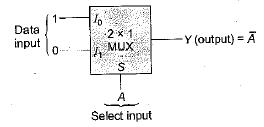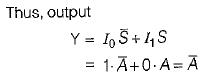Hence, statement-1 is not correct.

Statement-2 is correct because the internal circuit for a DEMUX (data distributor) and a decoder are basically same.

QUESTION: 2

### Match List-I (Combinational Circuits) with List-ll (Applications) and select the correct answer using the codes given below the lists: List-I A. Decoder B. Multiplexer C. Encoder D. Demultiplexer List-ll 1. Serial to parallel converter 2. Analog to digital converter 3. Parallel to serial converter 4. Digital to analog converter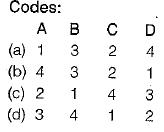Solution:

Decoder is a circuit which converts the digital signal into analog signal. Its input will be in digital form while the output will be a continuous sine wave or analog wave.
Encoders are combinational logic circuits and they are exactly opposite of decoders. They accept one or more inputs and generate a multi bit output code. Encoders perform exactly reverse operation than decoders. i.e analog to digital.
Multiplexer is a data selector which takes several inputs and gives a single output.In multiplexer we have 2n input lines and 1 output lines where n is the number of selection lines.
Demultiplexer is a data distributor which takes a single input and gives several outputs.In demultiplexer we have 1 input and 2n output lines where n is the selection line.

QUESTION: 3

### 7 The logic function F(A, B, 0 = 2(1,3,5, 6) can be implemented using:

Solution:

Given function,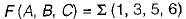has three variables. Hence, it can be implemented using a multiplexer ( 4 x 1 M U X) with two select inputs and four data inputs.
The implementation table is shown below: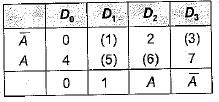Now, the given three variable functions can be implemented using 4-to-1 multiplexer as shown below: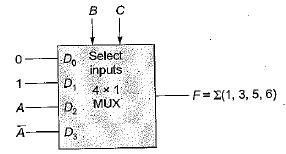Hence, for implementation of given function we require one 4 x 1 MUX and a NOT gate

QUESTION: 4

In a binary adder with two inputs X and Y, the correct set of logical expression for the output S (sum) and C (carry) are respectively

Solution:

The truth table for half adder is shown below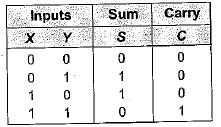Here, sum output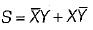and carry output is C= XY

QUESTION: 5

A certain multiplexer can switch one of 32 data inputs to its output. The number of control inputs in this multiplexer

Solution:

No. of input lines = 2m (where, m = No. of select/ control inputs) or, 32 = 2m or m=5.

QUESTION: 6

Consider the following statements associated with the use of various adder circuits:
1. A ripple carry adder is a parallel adder in which the carry-out of each full-adder is the carry-in to the next most significant adder.
2. Serial adders are used where speed is more important than circuit minimization.
3. The look-ahead carry adder speeds up the process by eliminating the ripple carry.
4. Serial adders are faster than parallel adders

Which of the statements given above are correct?

Solution:
• Serial adders are used where circuit minimization is more important than speed because they require one clock pulse for each pair of bits added and hence slower than parallel adders. Thus, statements 2 and 4 are not correct.
• Statements 1 and 3 are correct.
QUESTION: 7

Assertion (A): The multiplexer can be viewed as a function generator.
Reason (R): The multiplexer acts like a digitally controlled multi-position switch

Solution:
• The multiplexer can be viewed as a function generator because we can easily set or change the logic function it implements.
• The multiplexer acts like a digitally controlled multi-position switch because the digital code applied to the SELECT inputs determine which data inputs will be switched to the output.

Hence, both assertion and reason are correct but reason is not the correct explanation of assertion.

QUESTION: 8

Assertion (A): Using a decoder could have advantages over using a multiplexer.
Reason (R): The decoder is more economical in cases where non-trivial, multiple-output expressions of the same input variables are required

Solution:

In cases where non-trivial, a multiple-output expressions of the same input variables are required, a decoder could be advantageous than a multiplexer because in such cases, one multiplexer is required for each output, whereas it is likely that only one decoder supported with a few gates would be required.

QUESTION: 9

Assertion (A): The speed of operation of the parallel binary adder is high.

Reason (R): The parallel binary adder is said to generate its output immediately after the inputs are applied.

Solution:

The speed of operation of the parallel binary adder is limited by the carry propagation delay through all stages. Hence, assertion is not correct.

QUESTION: 10

A multiplexer has

Solution: